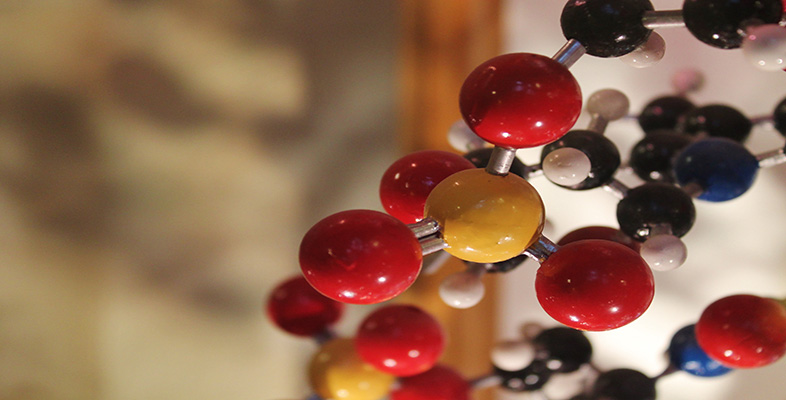Basic science: understanding numbers

Start this free course now. Just create an account and sign in. Enrol and complete the course for a free statement of participation or digital badge if available.

Free course

# 3.4 Fractions and PercentagesFigure 4

This week, you’ve seen how you may need to manipulate the bare numbers you obtain from an experiment in order to put them into a more useful format. As you saw in the bottled water video in Week 1, fractions and percentages are often used to communicate numbers in print, online and other audio-visual media. These are two simple techniques that you can use to make numbers more meaningful and they are not difficult to use.

## Fractions

Fractions are something which spark fear in a great number of people, yet simple fractions like a half (½) or a quarter (¼) are used every day. Numerically these two fractions are telling you how many of something you have (top number, or numerator) out of the total (bottom number, or denominator). For example, one out of every two hospital beds around the world is estimated to be occupied by someone suffering from a water-related illness. Using a fraction here provides much more context than an absolute number of beds occupied by those suffering from water-related illnesses without knowing the total number of beds.

Fractions do not need to be any more complicated than this. The thing that complicates fractions is that they are typically reported in their simplest form, meaning that they are converted such that their top and bottom numbers are as small as they can be while maintaining their proportions. This is so that they are easier to comprehend. For example, if a jar contained 900 sweets of which 300 were blue, this could be expressed as 300/900. Although this is correct, it’s common practice to reduce this to smaller numbers – otherwise known as ‘cancelling down’. In this example, both the top and bottom number can be dived by 300 resulting in the fraction ⅓ (called a ‘third’).

Scientists rarely present their results as a fraction because they can be fiddly to reduce to their smallest parts, and even when this is achieved the bottom number may differ, making it more difficult to compare like with like. Instead scientists commonly write fractions out as decimal numbers or percentages to communicate numbers as a proportion of the whole.

## Percentages

Take our example from earlier, where the scientist measured the amount of water in three different fruits. Their results indicated that 160 g of watermelon contained 144 g of water, 128 g of cucumber contained 120 g of water and 64 g of peach contained 56 g of water. Note that these weights have been rounded to the nearest whole number.

Percentages are calculated by dividing the number of parts you are interested in by the total number of parts available and multiplying the answer by 100. So, in the fruit example you might want to find out what percentage of the fruit is water. For a watermelon, you need to divide 144 by 160 and multiply the answer by 100. Try using your calculator to find the percentage. You should find the answer to be 90%.

### Activity 3.1 Calculating a percentage

Try calculating the percentage of water contained in the cucumber and the peach. Which of the fruits has the highest percentage of water content?

Percentages are not just used in science. You will have met percentages in other contexts – if you have received a 3% pay rise or seen an advert for a 20% off sale, for example. The same principles apply to these numbers as the fruit. For example, to calculate your pay following a 3% pay rise, you first calculate 3/100 of your income, and then add this result to your current annual income.

You can also think of this as 103% of your current salary. To work this out using your calculator or smartphone, multiply your current salary by 1.03. (Remember, your base salary is 100%.)

Some calculators and smartphones have a % button and you may have noticed that we haven’t used it during this section. That might seem a little odd but the reality is that we’re not sure that all % buttons operate consistently and we ultimately don’t understand how some of them work. As a result, it is far easier to simply ignore the existence of the % button entirely and just work out the percentage yourself.

Finally, the really scary stuff is when you try to add, subtract, multiply and divide fractions. The honest truth is scientists just convert these to decimal numbers and use a calculator.